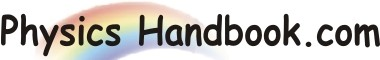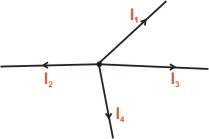HOME TOPICS DEFINITIONS TABLES LAWS INVENTIONS EXPERIMENTS QUIZ VIDEOS
 Kirchhoff's Current Law (KCL) The algebraic sum of the currents meeting at a junction in an electrical circuit is zero. An algebraic sum is one in which the sign of the quantity is taken into account.For example, consider four conductors carrying currents I1, I2, I3 and I4 and meeting at point O as shown in diagram given above. If we take the signs of currents flowing away form point O will be assigned negative sign. Thus, applying Kirchhoff's current law to the junction O in figure, we have ( I1 ) + ( I4 ) + ( -I2 ) + ( -I3 ) = 0 Or I1 + I4 = I2 + I3 i.e. sum of the incoming currents = sum of outgoing currents Hence Kirchhoff's current law may also be state as under : The sum of currents flowing towards any junction in an electrical circuit is equal to the sum of currents flowing away from that junction. Kirchhoff's current law is rightly called the junction rule. Kirchhoff's current law is true because electric current is merely the flow of free electrons and they cannot accumulate at any point in the circuit. This is in accordance with the law of conservation of charge. Hence Kirchhoff's current law is based on the law of conservation of charge.